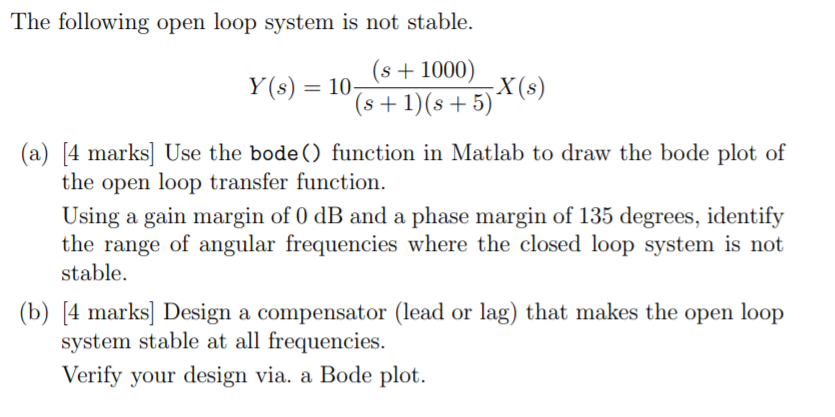# Question Control Systems Matlab Simulink The following open loop system is not stable. (s + 1000) Y(s) = 10 s (a) [4 marks] Use the bode () function in Matlab to draw the bode plot of the open loop transfer function. Using a gain margin of 0 dB and a phase margin of 135 degrees, identify the range of angular frequencies where the closed loop system is not stable. (b) [4 marks] Design a compensator (lead or lag) that makes the open loop system stable at all frequencies. Verify your design via. a Bode plot.BAPX95 The Asker · Electrical Engineering

Control Systems